### 5.6  Module Complex

Complex numbers.

This module provides arithmetic operations on complex numbers. Complex numbers are represented by their real and imaginary parts (cartesian representation). Each part is represented by a double-precision floating-point number (type `float`).

type (#'a:level) t = { re : 'a float; im : 'a float; }
The type of complex numbers. `re` is the real part and `im` the imaginary part.

val zero : 'a t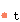The complex number `0`.

val one : 'a tThe complex number `1`.

val i : 'a tThe complex number `i`.

val neg : 'a t -> 'a t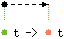Unary negation.

val conj : 'a t -> 'a tConjugate: given the complex `x + i.y`, returns `x - i.y`.

val add : 'a t -> 'a t -> 'a t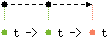val sub : 'a t -> 'a t -> 'a tSubtraction

val mul : 'a t -> 'a t -> 'a tMultiplication

val inv : 'a t -> 'a tMultiplicative inverse (`1/z`).

val div : 'a t -> 'a t -> 'a tDivision

val sqrt : 'a t -> 'a tSquare root. The result `x + i.y` is such that `x > 0` or `x = 0` and `y >= 0`. This function has a discontinuity along the negative real axis.

val norm2 : 'a t -> 'a floatNorm squared: given `x + i.y`, returns `x^2 + y^2`.

val norm : 'a t -> 'a float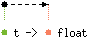Norm: given `x + i.y`, returns `sqrt(x^2 + y^2)`.

val arg : 'a t -> 'a floatArgument. The argument of a complex number is the angle in the complex plane between the positive real axis and a line passing through zero and the number. This angle ranges from `-pi` to `pi`. This function has a discontinuity along the negative real axis.

val polar : 'a float -> 'a float -> 'a t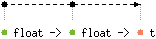`polar norm arg` returns the complex having norm `norm` and argument `arg`.

val exp : 'a t -> 'a tExponentiation. `exp z` returns `e` to the `z` power.

val log : 'a t -> 'a tNatural logarithm (in base `e`).

val pow : 'a t -> 'a t -> 'a tPower function. `pow z1 z2` returns `z1` to the `z2` power.